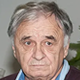## Joint Chinese-Russian Mathematical Online Colloquium

##### 11:00 (GMT+3)Jiajun Wang Peking University Bio: Jiajun Wang is a Professor and Head of the Department of Geometry and Topology at the School of Mathematical Sciences, Peking University. He received bachelor’s degree from Peking University in 2001 and PhD from UC Berkeley in 2007. Prof. Wang’s research interests are low-dimensional topology and gauge field.

### Fundamental Group and Heegaard Floer Homology.

Heegaard Floer homology and Monopole Floer Homology play an important role in the study of low dimensional topology. A three-manifold is essentially determined by its fundamental group. Ozsvath and Szabo asked for a concrete relation between the fundamental group and Heegaard Floer homology. We will talk about such a concrete relation for (1,1)-knots. This is joint work with Xiliu Yang.

##### 12:00 (GMT+3)Nikolay P. Dolbilin Steklov Mathematical Institute of RAS Bio: Nikolay Dolbilin is a leading researcher at the Steklov Mathematical Institute of the Russian Academy of Sciences and a part-time professor at Moscow State University. He also had long-term professor positions in mathematical centers and universities in Hungary, Germany, Japan, USA. Among scientific results are the following: the proof of a local criterion of regularity and development of the local theory of regular systems, the first complete proof of the celebrated Kac-Ward formula for the statistical sum for the Ising model, studies on the theory of parallelohedra (the concepts of the contact face and its index, a theorem on the sum of indices of contact faces). Besides, Nikolay Dolbilin paid much attention to the issues of mathematical education. He was an invited speaker at ICME-2000 at Tokyo/Makuhari, a member of the International Programm Committee of ICME-2004 in Copenhagen, a member of EC ICMI in 2003-2007.

### Local Conditions of Crystal Structures.

We will give an overview of the Local Theory of regular systems and also its connection with studies of quasicrystals and of arbitrary Delone/Delaunay set. The Local Theory of regular systems relates to the foundations of Geometric Crystallography.

The mathematical model of an ideal crystal (its atomic structure) is a discrete subset X in a finite-dimensional Euclidean space that is invariant with respect to some crystallographic group G of isometries of the Euclidean space. In other words, a crystal X is the union of a finite number of G-orbits.

A single-point orbit is termed a regular system. Our attention will be focused on the lower and upper bounds for the regularity radius, which is the minimum size of clusters whose pairwise equivalence at all points of a Delone set provides the regularity of the set.

The meeting will be held in the form of a webinar on the Zoom platform.

Pre-registration for the event is not required.